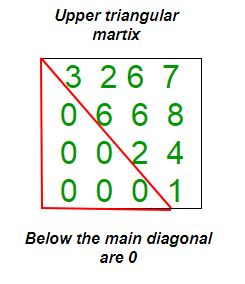# Program to check if matrix is upper triangular

Given a square matrix and the task is to check the matrix is in upper triangular form or not. A square matrix is called upper triangular if all the entries below the main diagonal are zero.Examples:

```Input : mat = {{1, 3, 5, 3},
{0, 4, 6, 2},
{0, 0, 2, 5},
{0, 0, 0, 6}};
Output : Matrix is in Upper Triangular form.

Input : mat = {{5, 6, 3, 6},
{0, 4, 6, 6},
{1, 0, 8, 5},
{0, 1, 0, 6}};
Output : Matrix is not in Upper Triangular form.
```

## C++

 `// Program to check upper triangular matrix. ` `#include ` `#define N 4 ` `using` `namespace` `std; ` ` `  `// Function to check matrix is in upper triangular ` `// form or not. ` `bool` `isUpperTriangularMatrix(``int` `mat[N][N]) ` `{ ` `    ``for` `(``int` `i = 1; i < N; i++) ` `        ``for` `(``int` `j = 0; j < i; j++) ` `            ``if` `(mat[i][j] != 0) ` `                ``return` `false``; ` `    ``return` `true``; ` `} ` ` `  `// Driver function. ` `int` `main() ` `{ ` `    ``int` `mat[N][N] = { { 1, 3, 5, 3 }, ` `                      ``{ 0, 4, 6, 2 }, ` `                      ``{ 0, 0, 2, 5 }, ` `                      ``{ 0, 0, 0, 6 } }; ` `    ``if` `(isUpperTriangularMatrix(mat)) ` `        ``cout << ``"Yes"``; ` `    ``else` `        ``cout << ``"No"``; ` `    ``return` `0; ` `} `

## Java

 `// Java Program to check upper  ` `// triangular matrix. ` `import` `java.util.*; ` `import` `java.lang.*; ` ` `  `public` `class` `GfG ` `{ ` `    ``private` `static` `final` `int` `N = ``4``; ` ` `  `    ``// Function to check matrix is in ` `    ``// upper triangular form or not. ` `    ``public` `static` `Boolean isUpperTriangularMatrix(``int` `mat[][]) ` `    ``{ ` `        ``for` `(``int` `i = ``1``; i < N ; i++) ` `            ``for` `(``int` `j = ``0``; j < i; j++) ` `                ``if` `(mat[i][j] != ``0``) ` `                    ``return` `false``; ` `        ``return` `true``; ` `    ``}  ` `     `  `    ``// driver function ` `    ``public` `static` `void` `main(String argc[]){ ` `        ``int``[][] mat= { { ``1``, ``3``, ``5``, ``3` `}, ` `                       ``{ ``0``, ``4``, ``6``, ``2` `}, ` `                       ``{ ``0``, ``0``, ``2``, ``5` `}, ` `                       ``{ ``0``, ``0``, ``0``, ``6` `} }; ` `                     `  `        ``if` `(isUpperTriangularMatrix(mat)) ` `            ``System.out.println(``"Yes"``); ` `        ``else` `            ``System.out.println(``"No"``); ` `    ``} ` `} ` ` `  `/* This code is contributed by Sagar Shukla */`

## Python3

 `# Python3 Program to check upper  ` `# triangular matrix. ` ` `  `# Function to check matrix  ` `# is in upper triangular ` `def` `isuppertriangular(M): ` `    ``for` `i ``in` `range``(``1``, ``len``(M)): ` `        ``for` `j ``in` `range``(``0``, i): ` `            ``if``(M[i][j] !``=` `0``):  ` `                    ``return` `False` `    ``return` `True` `     `  `# Driver function. ` `M ``=` `[[``1``,``3``,``5``,``3``], ` `    ``[``0``,``4``,``6``,``2``], ` `    ``[``0``,``0``,``2``,``5``], ` `    ``[``0``,``0``,``0``,``6``]] ` ` `  `if` `isuppertriangular(M): ` `    ``print` `(``"Yes"``) ` `else``: ` `    ``print` `(``"No"``) ` ` `  `# This code is contributed by Anurag Rawat `

## C#

 `// C# Program to check upper  ` `// triangular matrix. ` `using` `System; ` ` `  `public` `class` `GfG ` `{ ` `    ``private` `static` `int` `N = 4; ` ` `  `    ``// Function to check matrix is in ` `    ``// upper triangular form or not. ` `    ``public` `static` `bool` `isUpperTriangularMatrix(``int` `[,]mat) ` `    ``{ ` `        ``for` `(``int` `i = 1; i < N ; i++) ` `            ``for` `(``int` `j = 0; j < i; j++) ` `                ``if` `(mat[i, j] != 0) ` `                    ``return` `false``; ` `        ``return` `true``; ` `    ``}  ` `     `  `    ``// Driver function ` `    ``public` `static` `void` `Main(){ ` `        ``int` `[,]mat= { { 1, 3, 5, 3 }, ` `                    ``{ 0, 4, 6, 2 }, ` `                    ``{ 0, 0, 2, 5 }, ` `                    ``{ 0, 0, 0, 6 } }; ` `                     `  `        ``if` `(isUpperTriangularMatrix(mat)) ` `            ``Console.WriteLine(``"Yes"``); ` `        ``else` `            ``Console.WriteLine(``"No"``); ` `    ``} ` `} ` ` `  `/* This code is contributed by vt_m */`

## PHP

 ` `

Output:

`Yes`

Don’t stop now and take your learning to the next level. Learn all the important concepts of Data Structures and Algorithms with the help of the most trusted course: DSA Self Paced. Become industry ready at a student-friendly price.

My Personal Notes arrow_drop_upCheck out this Author's contributed articles.

If you like GeeksforGeeks and would like to contribute, you can also write an article using contribute.geeksforgeeks.org or mail your article to contribute@geeksforgeeks.org. See your article appearing on the GeeksforGeeks main page and help other Geeks.

Please Improve this article if you find anything incorrect by clicking on the "Improve Article" button below.

Improved By : vt_m

Article Tags :
Practice Tags :

1

Please write to us at contribute@geeksforgeeks.org to report any issue with the above content.